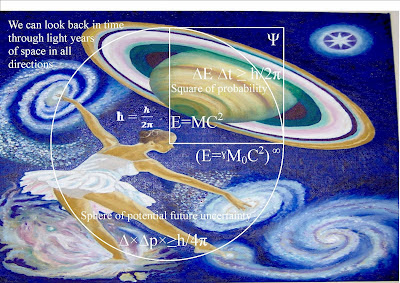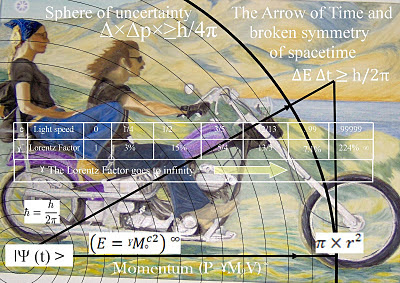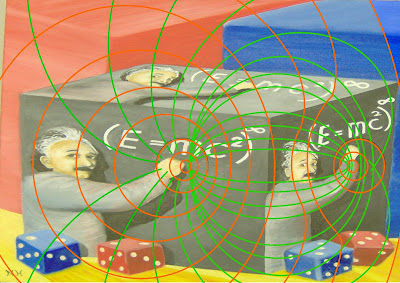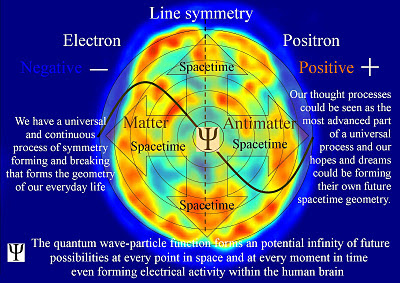Tuesday, 7 February 2012

Quantum Atom Theory an artist interpretation of quantum mechanicsQuantum Atom Theory is an artist theory it is a visual theory based on observations not mathematics. So I have used the equations of Einstein and quantum physics and looked at them as a kind of abstract art. They are very beautiful looked at this way.This equation is a set of infinities within infinity a time within a spacetime a rhyme within a rhyme.
~

Above is Quantum Atom Theory interpretation of Einstein famous equation E=MC2. The (E) energy = (ˠ) Lorentz factor (M0) rest mass times the square of the speed of light (C2). The brackets represent the boundary condition of an individual reference frame. The (∞) infinity sign represents the infinity of an infinite number of inertial and non-inertial reference frames.

The big different with (E=ˠM0C2) ∞ and the original E=MC2 is that the brackets represent a boundary condition of a reference frame.

The conditions (rate that time ∆t flows) within each reference frames will be relative to the energy ∆E of that reference frame.

This theory explains how time ∆E ∆t ≥ h/2π is formed by the quantum wave particle function Ψ expanding out from its radius forming a sphere 4π in three dimensional space. The surface area of the sphere will form the two dynamic dimensions 2π of a past and a potential uncertain future ∆×∆p×≥h/4π of position and momentum.

In this theory the quantum wave particle function Ψ is not just a function of time ∆E ∆t ≥ h/2π it represents the forward passage of time itself photon by photon.

Each new photon-oscillation is a new moment in time that will have the three dimensions of spacetime.

Therefore Heisenberg’s Uncertainty Principle ∆×∆p×≥h/4π that is formed by the quantum wave particle function Ψ is the same uncertainty we have with any future event.

In Quantum Atom Theory the atoms interact with the wave particle duality of light. Because we are made of atoms our position and momentum will affect where and when the w-function collapses and therefore how the future unfolds.

We have free-will to manipulate the atoms within an individual reference frame changing the probability of a potential future outcome.

Therefore we have the freedom to create art and poetry!

In this theory the thing driving future moments is “stimulated emission” The spontaneous emission of light forms the flow of time forming greater degrees of freedom for the flow of statistical entropy.

The 2nd law of thermodynamics falls out of this theory the organization for the spontaneous disorganization of entropy is formed by the spherical 4π symmetry of the wave function. The spontaneous emission of light forms the flow of time forming greater degrees of freedom for the flow of entropy.
~

Wednesday, 1 February 2012

Quantum Atom Theory is an artist theory on the physics of time as a physical processQuantum Atom Theory is an artist theory on the physics of time as a physical process of continuous energy exchange continuous change. This process “stimulated emission” is formed by the spontaneous emission and absorption of light.When there is an absorption and emission of light we have a quantum wave particle function that forms the uncertainty of Heisenberg’s Uncertainty Principle ∆×∆p×≥h/4π.

In this theory Heisenberg’s Uncertainty Principle ∆×∆p×≥h/4π is the same uncertainty we have with any future event and the quantum wave particle function represents part of a physical process that we see and feel as the flow of ∆E ∆t ≥ h/2π time itself.We have an infinite number of reference frames within our Universe and because light has momentum and momentum is frame dependent each object or observer will have their own uncertainty as time unfolds photon by photon.An observer can look back in time at the beauty of the stars in all directions from the centre of their own reference frame. This is because they are forming their own spacetime by collapsing the waves of light into new photon-oscillations forming their own future potential.

This is a continuous dynamic process in three dimensional space the wave function will expand out from its radius forming a sphere 4π.

It is a probability wave of a potential future event and during the act of measurement the magnitude squared C2 gives a probability for different potential future outcomes.The surface area of the sphere 4π forms a two 2π dimensional boundary condition that we see and feel as the two dynamic dimensions of a past and future with potential uncertainty ∆×∆p×≥h/4π formed by the flow of time ∆t.This forms the continuous flow of electric charge with the movement of electromagnetic fields the electric fields will always be at right angles to the magnetic fields forming the local spacetime geometry.Consciousness the continuous flow of ideas formed by electrical activity within the brain is the most advanced part of this universal process and can therefore understand it as the flow of time with a past and a potential future.This whole process can be seen as a process of symmetry forming and breaking between matter and antimatter. In this theory antimatter annihilation is part of the process that forms the flow of time.

We have a dynamic Universe of continuous change continuous creation forming the uniqueness of every moment.

~